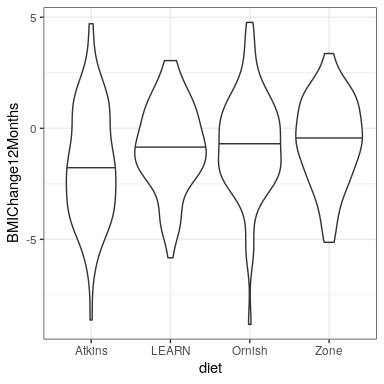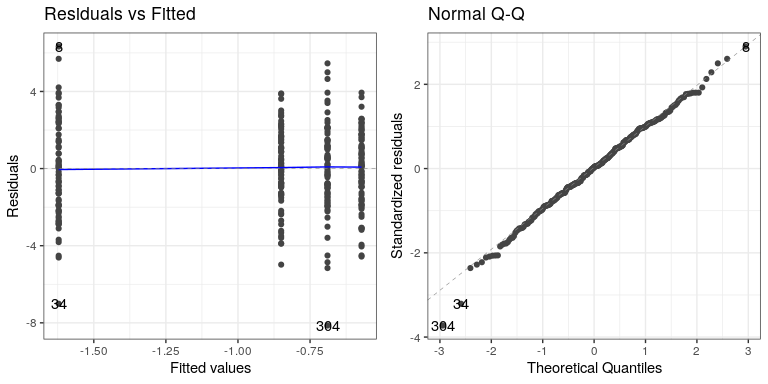# 30 Practical statistical modeling

In this chapter we will bring together everything that we have learned to apply our knowledge to a practical example.

## 30.1 The process of statistical modeling

There is a set of steps that we generally go through when we want to use our statistical model to test a scientific hypothesis:

1. Specify your question of interest
2. Identify or collect the appropriate data
3. Prepare the data for analysis
4. Determine the appropriate model
5. Fit the model to the data
6. Criticize the model to make sure it fits properly
7. Test hypothesis and quantify effect size

Let’s look at a real example. In 2007, Christopher Gardner and colleagues from Stanford published a study in the Journal of the American Medical Association titled “Comparison of the Atkins, Zone, Ornish, and LEARN Diets for Change in Weight and Related Risk Factors Among Overweight Premenopausal Women The A TO Z Weight Loss Study: A Randomized Trial” (Gardner et al. 2007).

### 30.1.1 1: Specify your question of interest

According to the authors, the goal of their study was:

To compare 4 weight-loss diets representing a spectrum of low to high carbohydrate intake for effects on weight loss and related metabolic variables.

### 30.1.2 2: Identify or collect the appropriate data

To answer their question, the investigators randomly assigned each of 311 overweight/obese women to one of four different diets (Atkins, Zone, Ornish, or LEARN), and measured their weight and other measures of health over time.

The authors recorded a large number of variables, but for the main question of interest let’s focus on a single variable: Body Mass Index (BMI). Further, since our goal is to measure lasting changes in BMI, we will only look at the measurement taken at 12 months after onset of the diet.

### 30.1.3 3: Prepare the data for analysisFigure 30.1: Violin plots for each condition, with the 50th percentile (i.e the median) shown as a black line for each group.

The actual data from the A to Z study are not publicly available, so we will use the summary data reported in their paper to generate some synthetic data that roughly match the data obtained in their study. Once we have the data, we can visualize them to make sure that there are no outliers. Violin plots are useful to see the shape of the distributions, as shown in Figure 30.1. Those data look fairly reasonable - in particular, there don’t seem to be any serious outliers. However, we can see that the distributions seem to differ a bit in their variance, with Atkins and Ornish showing greater variability than the others.

This means that any analyses that assume the variances are equal across groups might be inappropriate. Fortunately, the ANOVA model that we plan to use is fairly robust to this.

### 30.1.4 4. Determine the appropriate model

There are several questions that we need to ask in order to determine the appropriate statistical model for our analysis.

• What kind of dependent variable?
• BMI : continuous, roughly normally distributed
• What are we comparing?
• mean BMI across four diet groups
• ANOVA is appropriate
• Are observations independent?
• Random assignment and use of difference scores should ensure that the assumption of independence is appropriate

### 30.1.5 5. Fit the model to the data

Let’s run an ANOVA on BMI change to compare it across the four diets. It turns out that we don’t actually need to generate the dummy-coded variables ourselves; if we pass lm() a categorical variable, it will automatically generate them for us.

##
## Call:
## lm(formula = BMIChange12Months ~ diet, data = dietDf)
##
## Residuals:
##    Min     1Q Median     3Q    Max
##  -8.14  -1.37   0.07   1.50   6.33
##
## Coefficients:
##             Estimate Std. Error t value Pr(>|t|)
## (Intercept)   -1.622      0.251   -6.47  3.8e-10 ***
## dietLEARN      0.772      0.352    2.19   0.0292 *
## dietOrnish     0.932      0.356    2.62   0.0092 **
## dietZone       1.050      0.352    2.98   0.0031 **
## ---
## Signif. codes:  0 '***' 0.001 '**' 0.01 '*' 0.05 '.' 0.1 ' ' 1
##
## Residual standard error: 2.2 on 307 degrees of freedom
## Multiple R-squared:  0.0338, Adjusted R-squared:  0.0243
## F-statistic: 3.58 on 3 and 307 DF,  p-value: 0.0143

Note that lm automatically generated dummy variables that correspond to three of the four diets, leaving the Atkins diet without a dummy variable. This means that the intercept models the Atkins diet, and the other three variables model the difference between each of those diets and the Atkins diet. By default, lm() treats the first value (in alphabetical order) as the baseline.

### 30.1.6 6. Criticize the model to make sure it fits properlyThe first thing we want to do is to critique the model to make sure that it is appropriate. One thing we can do is to look at the residuals from the model. In the left panel of Figure ??, we plot the residuals for each individual grouped by diet, which are positioned by the mean for each diet. There are no obvious differences in the residuals across conditions, although there are a couple of datapoints (#34 and #304) that seem to be slight outliers.

Another important assumption of the statistical tests that we apply to linear models is that the residuals from the model are normally distributed. The right panel of Figure ?? shows a Q-Q (quantile-quantile) plot, which plots the residuals against their expected values based on their quantiles in the normal distribution. If the residuals are normally distributed then the data points should fall along the dashed line — in this case it looks pretty good, except for those two outliers that are once again apparent here.

### 30.1.7 7. Test hypothesis and quantify effect size

First let’s look back at the summary of results from the ANOVA, shown in Step 5 above. The significant F test shows us that there is a significant difference between diets, but we should also note that the model doesn’t actually account for much variance in the data; the R-squared value is only 0.03, showing that the model is only accounting for a few percent of the variance in weight loss. Thus, we would not want to overinterpret this result.

The significant result also doesn’t tell us which diets differ from which others. We can find out more by comparing means across conditions using the emmeans() (“estimated marginal means”) function:

##  diet   emmean    SE  df lower.CL upper.CL .group
##  Atkins  -1.62 0.251 307    -2.11    -1.13  a
##  LEARN   -0.85 0.247 307    -1.34    -0.36  ab
##  Ornish  -0.69 0.252 307    -1.19    -0.19   b
##  Zone    -0.57 0.247 307    -1.06    -0.08   b
##
## Confidence level used: 0.95
## P value adjustment: tukey method for comparing a family of 4 estimates
## significance level used: alpha = 0.05

The letters in the rightmost column show us which of the groups differ from one another, using a method that adjusts for the number of comparisons being performed. This shows that Atkins and LEARN diets don’t differ from one another (since they share the letter a), and the LEARN, Ornish, and Zone diets don’t differ from one another (since they share the letter b), but the Atkins diet differs from the Ornish and Zone diets (since they share no letters).

#### 30.1.7.1 Bayes factor

Let’s say that we want to have a better way to describe the amount of evidence provided by the data. One way we can do this is to compute a Bayes factor, which we can do by fitting the full model (including diet) and the reduced model (without diet) and then comparing their fit. For the reduced model, we just include a 1, which tells the fitting program to only fit an intercept. Note that this will take a few minutes to run.

This shows us that there is very strong evidence (Bayes factor of nearly 100) for differences between the diets.

### 30.1.8 What about possible confounds?

If we look more closely at the Garder paper, we will see that they also report statistics on how many individuals in each group had been diagnosed with metabolic syndrome, which is a syndrome characterized by high blood pressure, high blood glucose, excess body fat around the waist, and abnormal cholesterol levels and is associated with increased risk for cardiovascular problems. Let’s first add those data into the summary data frame:

Table 30.1: Presence of metabolic syndrome in each group in the AtoZ study.
Diet N P(metabolic syndrome)
Atkins 77 0.29
LEARN 79 0.25
Ornish 76 0.38
Zone 79 0.34

Looking at the data it seems that the rates are slightly different across groups, with more metabolic syndrome cases in the Ornish and Zone diets – which were exactly the diets with poorer outcomes. Let’s say that we are interested in testing whether the rate of metabolic syndrome was significantly different between the groups, since this might make us concerned that these differences could have affected the results of the diet outcomes.

#### 30.1.8.1 Determine the appropriate model

• What kind of dependent variable?
• proportions
• What are we comparing?
• proportion with metabolic syndrome across four diet groups
• chi-squared test for goodness of fit is appropriate against null hypothesis of no difference

Let’s compute that statistic using the chisq.test() function. Here we will use the simulate.p.value option, which will help deal with the relatively small

##
##  Pearson's Chi-squared test
##
## data:  contTable
## X-squared = 4, df = 3, p-value = 0.3

This test shows that there is not a significant difference between means. However, it doesn’t tell us how certain we are that there is no difference; remember that under NHST, we are always working under the assumption that the null is true unless the data show us enough evidence to cause us to reject this null hypothesis.

What if we want to quantify the evidence for or against the null? We can do this using the Bayes factor.

## Bayes factor analysis
## --------------
##  Non-indep. (a=1) : 0.058 ±0%
##
## Against denominator:
##   Null, independence, a = 1
## ---
## Bayes factor type: BFcontingencyTable, independent multinomial

This shows us that the alternative hypothesis is 0.058 times more likely than the null hypothesis, which means that the null hypothesis is 1/0.058 ~ 17 times more likely than the alternative hypothesis given these data. This is fairly strong, if not completely overwhelming, evidence.